#The Wolfram Language:Fast Introduction for Programmers

Show additional notes for:
All sectionsGet Started

# Interactive InterfacesView as Video

You can take any expression and "manipulate" it with controls that change values of variables:

In:=1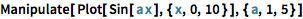`Manipulate[Plot[Sin[a x], {x, 0, 10}], {a, 1, 5}]`
Out=1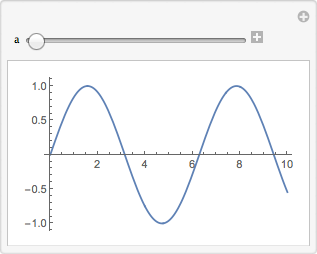Notes for Java programmers:

The Manipulate command greatly simplifies GUI creation, automatically inferring control types and layout based on parameter specifications. Interface creation in Java requires the use of Swing, AWT or a third-party GUI library, typically with many more lines of code.

Notes for Python programmers:

Manipulate in the Wolfram Language greatly simplifies GUI creation, automatically inferring control types and layout based on parameter specifications, and runs seamlessly on desktop, cloud and mobile devices using the exact same code. In Python, building interactive interfaces requires custom code for a standalone GUI or using a third-party notebook library. Creating the same interface in Python for the desktop and cloud requires writing separate code for both platforms.

Manipulate uses the standard min, max, step iterator specification:

In:=2`Manipulate[Range[n], {n, 4, 10, 1}]`
Out=2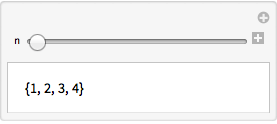In the Wolfram Language, controls are just symbolic expressions:

In:=1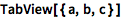`TabView[{a, b, c}]`
Out=1Make a button by giving an expression to evaluate when the button is pressed:

In:=2`Button["do it", Speak["hello"]]`
Out=2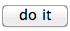QUICK REFERENCE: Interactive Controls

Dynamic displays as the dynamically updated value of an expression.
(This is always possible in local, desktop versions; support may be limited in the cloud.)

Move the slider to get the value of x to change:

In:=1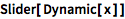`Slider[Dynamic[x]]`
Out=1Show the current value:

In:=2`x`
Out=2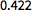Always display the current value:

In:=3`Dynamic[x]`
Out=3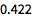Notes for Java programmers:

Dynamic is a powerful construct for tying values together and has no direct analog in Java. Java would require a method call, background threads or additional logic to update the display every time x changes.

Notes for Python programmers:

Dynamic is a powerful and unique Wolfram Language construct that dynamically updates its displayed output whenever there is a change, and it also forms the foundation for Manipulate. In Python, there is no automatic way to do this, but you can integrate Python code with the Wolfram Language using ExternalEvaluate.

QUICK REFERENCE: Interactive Interfaces

Which of the following makes a Manipulate with a slider for x from 1 to 4?

If one puts x = 2 in a first cell and Dynamic[x] in a second cell, then sets x = 4 in a third cell, what is in the output of the second cell after those three cells are evaluated?

Which of these gives a button that sets the value of x to 4?